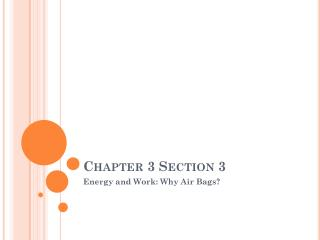DownloadDownload PresentationChapter 3 Section 3

# Chapter 3 Section 3

Télécharger la présentation## Chapter 3 Section 3

- - - - - - - - - - - - - - - - - - - - - - - - - - - E N D - - - - - - - - - - - - - - - - - - - - - - - - - - -
##### Presentation Transcript

1. Chapter 3 Section 3 Energy and Work: Why Air Bags?

2. Physics 3.3: Energy and WorkHomework 1-10, Skip 4 and 7 Due Friday • Learning Objective • Students will be able to describe the relationship between stopping distance and force required to stop an object • Success Criteria • Model an automobile air bag • Relate W=F·D to the eggsperiment (get it?) • Do Now: • QUIZ! • Agenda • Do Now • Investigate 3.3 • Summary/Exit Ticket

3. Egg Lab  • Dropping Eggs! • Throwing an egg in a blanket

4. Exit Ticket • How did the indentation of the egg in the flour change as you increased the initial drop height?

5. Physics Talk 3.3 • Learning Objective • Students will be able to explain the relationship between work and kinetic energy • Success Criteria • Students will be able to explain the relationship between stopping distance and force required to stop • Students will be able to explain the relationship between velocity and braking distance • Students will describe which features of a car help in the case of a collision • Do Now: • WDYS/WDYT p 277 • Agenda • Do Now • Physics Talk 3.3 • What do you think now? • Summary

6. Physics Talk 3.3 • What is kinetic energy? • What is the equation? • The energy an object has because of its motion.

7. Kinetic Energy and the Eggs • The two eggs were dropped from the same height. Which had more kinetic energy? • Since both eggs had approximately the same mass and same relative velocity, they about the same kinetic energy.

8. Measuring Force Over a Distance • How was the egg able to stop? • How do you measure the distance in each egg drop? • The surface had to apply a force over some distance. • When the egg was dropped in flour, you can measure the indentation. With the hard surface, you cannot see the indentation.

9. Braking a Car • How do auto brakes work? • The braking system has to eliminate the kinetic energy of the vehicle by applying a large force over a large distance.

10. Work • How do you calculate work? • How does work relate to kinetic energy? • W=F·D • Work is the change in kinetic energy. We can write this as

11. Braking a Car

12. Example

13. What about the eggs? • How does this apply to the eggs? • When the egg could be stopped over a greater distance, there was less damage to it because less force had to be applied.

14. How does this apply to a car crash? • Airbags! These allow you to have more distance to stop your body so you will be less likely to get hurt.

15. If you are traveling three-times the speed, will you need three times the braking distance? • False! You will need 9 times the stopping distance. This is because in the formula, the velocity is squared

16. Why is it harder to stop on icy or wet roads? • The slippery surface makes it more difficult to supply a sufficient force, so it is necessary to give yourself more distance.

17. What else helps stop an automobile? • The crumple zone of a car allows the car to have more distance to stop. This is why cars are designed to dent and are not made out of more sturdy material.

18. Sample Problem • p286 and 287 Sample Problem

19. What do you think now? Exit Ticket: How does and air bag protect you during an accident?